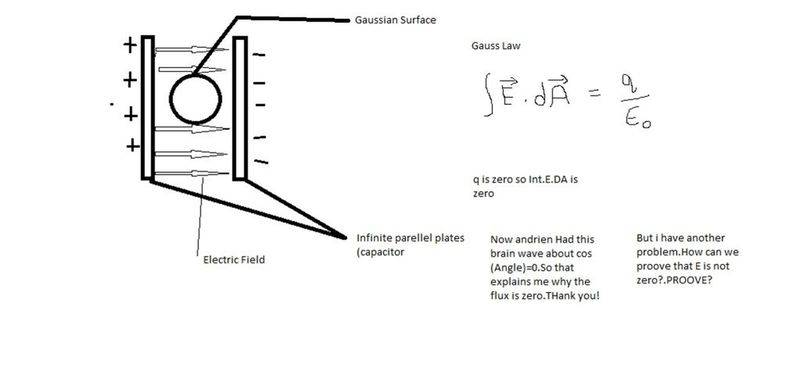# Electric field at point inside a parallel plate capacitor

This doubt is nagging in my mind for couple of days,and its makes the rest of the things so dizzy and confusing.Heres what i thought-

What is the electric field at a point inside a parallel plate capacitor?

I thought of this-When i place a guassian surface of ,well maybe any shape,between the parallel plates the electric field lines all pass through it,so net flux should be zero?and if it is zero E should also be zero?

But common sense tell me E can't be a zero,and something is wrong with all the above thinking.What can it be?

Also please explain in clear language,i googled and ended up in i don't know what,then i came here expecting help.

Aimless
If you make the approximation that the plates are infinite in size, then the electric field lines in the region between the two plates run perpendicular to the plates (parallel to each other) with constant field strength determined by the charge on the capacitor.

If the two plates aren't infinite in extent, there will be some bending of the field lines due to the edge effects, but this is usually negligible.

That i know,that wasn't my question.My question was-What if we draw a Gaussian surface BETWEEN the parallel plate capacitor,Why Electric field isn't zero?Why?

Staff Emeritus
Homework Helper
That i know,that wasn't my question.My question was-What if we draw a Gaussian surface BETWEEN the parallel plate capacitor,Why Electric field isn't zero?Why?

Welcome to Physics Forums.

There are two ways I can think of to explain why the flux is zero, even if the field isn't:

1. The flux due to field lines entering the surface is equal in magnitude to the flux due to field lines. These two effects cancel each other, resulting in zero total flux.

2. You're apparently familiar with Gauss's Law. Since there is no charge enclosed by the surface, the flux must be zero for the surface -- even if the field is not zero.

Hope that helps.

Ok so you just said it.Flux is zero.That means

Integration E.dA is zero!
Area can't be zero so Electric field is zero!But that can't be?How can electric field be zero between a parrallel plate capacitor?

andrien
that is not it's meaning.notice it is a dot product so it is proportional to cosine of the angle between normal and electric field direction .on one side it is positive and on the other it is negative so flux will become zero.Mentor
Ok so you just said it.Flux is zero.That means

Integration E.dA is zero!
Area can't be zero so Electric field is zero!But that can't be?How can electric field be zero between a parrallel plate capacitor?

At some points on the surface ${E}\bullet{A}$ is positive and at other points it's negative. So although E is not zero the integral still comes out zero when the positive and the negative values cancel.

Homework Helper
Gold Member
Ok so you just said it.Flux is zero.That means

Integration E.dA is zero!
Area can't be zero so Electric field is zero!But that can't be?How can electric field be zero between a parrallel plate capacitor?

Does $\int_a^b f(x) =0$ where $b>a$ imply that $f(x)=0$?...Of course not, as a counterexample consider $f(x)=\cos \left( \frac{\pi x}{b-a} \right)$.

Similarily, $\oint \mathbf{E} \cdot d\mathbf{A}=0$ does not mean that $\mathbf{E}$ is zero.

To prove (there is only one "o" in prove) that the electric field is not zero, you just need to calculate it. You can do so by applying Gauss' Law with a pillbox that straddles one plate, or by using Coulomb's law, or by solving Laplace's equation for the potential and then taking the negative gradient. There are many places on the internet where the calculation is shown in detail.